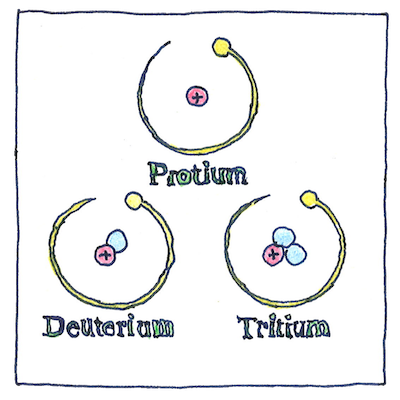# 1922

## The book of science

Tom Sharp

 Francis William Aston, Frederick Soddy chemistry

## Isotope

• Atomic masses
• seemed to vary
• with the source
• of the sample,
• and it irritated
• chemists
• to find masses
• that were not multiples
• of the mass
• of the hydrogen atom.
• *
• Francis William
• Aston improved
• the mass
• spectrograph
• and found neon ions
• (atom number 10)
• atomic masses
• nearly 20
• and nearly 22,
• in any event
• not the same
• as the atomic mass
• of neon gas,
• which led Aston
• to conclude
• measured masses
• were for
• a mixture
• of isotopes.

## Chain of decay

• Frederick Soddy introduced the idea of isotopes
• to explain the decay of radioactive elements.
• There are only nine elements
• in the periodic table between uranium and lead.
• but uranium decays into lead
• through chains of about forty particles,
• each distinguishable by its atomic mass
• but impossible to separate chemically.

## A problem

• What are these atoms
• chemically
• the same but
• have different
• masses
• from different sources?
• Are our methods
• too crude
• or is the element variable?

The number of protons in an atom, known as its atomic number, determine what element it is. An isotope is an atom with a different number of neutrons, and an ion is an atom with a different number of electrons.

Isotopes of the same element occupy the same place in the periodic table, which is why Frederick Soddy and Margaret Todd coined the term isotope, Greek for “at the same place.”

Atomic masses are measured in units where 1 is equal to the total mass of one proton, one neutron, and one electron in carbon-12.

The mass number of an atom is the sum of its protons and neutrons (both known as nucleons). The element name and mass number identify the isotope.

For example, the atomic number of carbon is 6, and the carbon-14 isotope, abbreviated as 14C, has 6 protons and 8 neutrons.

Only 80 elements have stable isotopes, the rest having only radioactive isotopes. Tin, atomic number 40, has the largest number of stable isotopes, ten, with mass numbers 112, 114 through 120, 122, and 124. Tin-124 has 40 protons and 84 neutrons.

If you are talking about various atoms that occupy the same place in the periodic table, you use the term isotope. If you are talking about various nuclei with the same number of protons, you use the term nuclide. It turns out to be an urban myth that Eskimos have 100 words for snow, but it is true that Americans have at least 100 words for stupidity.